Goseeko blog# What is a function?

Definition: Let X and Y are two sets. A relation f from X to Y is a function if for every x ∈ X there is a unique element y ∈ Y, such that (x, y) ∈ X

Here we denote it as f: X → Y, which means f is a function from X to Y.

Hence, We represent it as-

Example: Let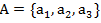And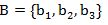And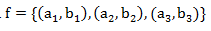Hence we represent the function as below

Here A is the domain of f and B is the co-domain of f

Surjective function (Onto-Mapping)

A mapping f: X → Y said to be onto-mapping if the range set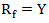.

If  f: X → Y is onto then each element of B if f-image of at least one element of X.

That means,

A mapping is a into if it is not onto.

## Injective fn (One-To-One Mapping)-

A mapping f: X → Y is said to be one-to-one mapping if distinct elements of X are mapped into distinct elements of Y.

That means if f is a one-to-one mapping, then-

Or in other words,

Bijective functions (One-To-One, Onto)-

A mapping f: X → Y is the bijective mapping if it is both one-to-one and onto.

Identity function (Identity Mapping)-

If f: X → Y  is a function such that every element of X is mapped onto itself then f is said to be an identity mapping.

We denote identity mapping by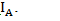Ifis an identity mapping.

Partial functions-

Let X and Y be sets and A be a subset of X. A function f from A to Y is called a partial func. from X to Y. The set A is a called the domain of f.

Constant function-

Let f: X → Y  then f is a constant function if every element of X is map onto the same element of Y.

Inverse functions-

Let f: X → Y is a one-one and onto mapping, then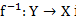is called the inverse mapping of f.

We define the Inverse mapping as

Composition of Functions

Let f: X → Y and g: Y → Z are two mappings, then we denote the composition of these two mappings f and g by gof is the mapping from X into Z.

Hence, we define it as

That means  is a mapping defined as below-

Invertible function-

A function f from a set X to a set Y is an invertible func if there exists a function g from Y to X, such that-

f(g(y)) = y And g(f(x)) = x For every x ∈ X and y∈ Y

Recursive Functions- A function f is a Primitive recursive if we obtain it from the initial functions by a finite number of operations of composition and recursion.

Interested in learning about similar topics? Here are a few hand-picked blogs for you!